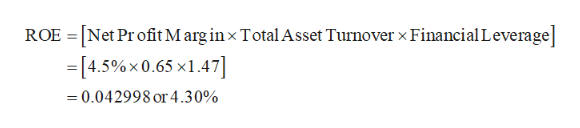# If we know that a firm has a net profit margin of 4.5 % total asset turnover of 0.65, and a financial leverage multiplier of 1.47, what is its​ ROE? What is the advantage to using the DuPont system to calculate ROE over the direct calculation of earnings available for common stockholders divided by common stock​ equity?

Question
50 views

If we know that a firm has a net profit margin of 4.5 % total asset turnover of 0.65, and a financial leverage multiplier of 1.47, what is its​ ROE? What is the advantage to using the DuPont system to calculate ROE over the direct calculation of earnings available for common stockholders divided by common stock​ equity?

check_circle

Step 1

Calculation of ROE:

The ROE is 4.30%.

...help_outlineImage TranscriptioncloseROE = [Net Pr ofit M arg inx Total Asset Turnover x Financial Leverage] [4.5%x 0.65 x1.47] 0.042998 or4.30% fullscreen

### Want to see the full answer?

See Solution

#### Want to see this answer and more?

Solutions are written by subject experts who are available 24/7. Questions are typically answered within 1 hour.*

See Solution
*Response times may vary by subject and question.
Tagged in